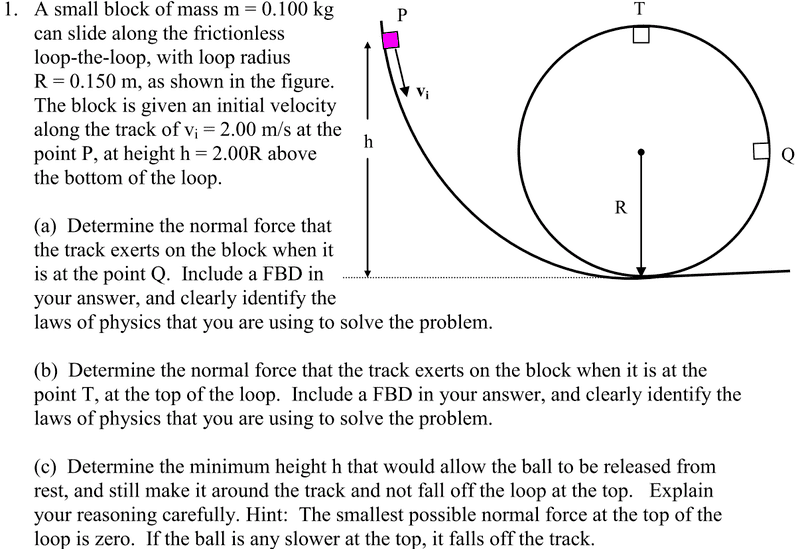# Small block on a track - Finding Normal forces and

• nukeman
The speed at point Q is 2.00 m/s.In summary, the question is asking for the forces at point Q, which include the force of gravity and the normal force. To perform circular motion, a mass needs a centripetal force towards the center of the circle, which is calculated using the formula F = mv^2/r. The speed at point Q can be found using conservation of energy principle.

#### nukeman

Small block on a track - Finding Normal forces and...

## Homework Statement

Here is the question. Can someone please help, since I missed the section of class that went over this, any my textbook does not cover this well. Look below at question 1, a) and b)## The Attempt at a Solution

Which are the forces at point Q?

At point Q - Forces are Gravity down... Does it have tangential and radial?

The block is touching the track. Hence besides the weight there is also the ...

grzz said:
The block is touching the track. Hence besides the weight there is also the ...

Force of gravity and the normal force

Correct.
Now for a mass to perform circular motion, it needs a ...

grzz said:
Correct.
Now for a mass to perform circular motion, it needs a ...

Force?

What do we call this particular force?

I am not sure. Momentum force?

Any mass performing circular motion needs a force towards the centre of the circle. This particular force is called ...

Centripetal Force?

CORRECT.

what is the formula for this centripetal force in terms of the speed at Q?

grzz said:
CORRECT.

what is the formula for this centripetal force in terms of the speed at Q?

I am not sure on that... I can't find it in my book (awfull book). :(

?

This forum is for helping and not for doing the work for you.
Try looking up 'centripeta force' on internet search engine. That is the way to learn.

Did not mean to imply that.

F = MAc = MV^2/r

Is that it? what is the c ?

It is ok. You are welcome to this forum!

You got the right formula.
A - acceleration, c- centripetal.

Now try to find the speed at point Q.

This is where i have trouble.

When i plug everything in:

F = (.100)(2.00)^2 / (.150)

I get 2.66 ?

Remember that we were talking about the mass when it is at point Q. So we are trying to find the centripetal force at point Q. But the speed you are using is 2m/s which is the speed at a higher point and not the speed at Q.

Try to find the speed at Q.

I don't understand how I would change that formula to find the centripetal force at point Q

The first part of the problem was to find the normal force at Q.

To do this we need to find the centripetal force at Q.

This centripetal force = mv$^{2}$/R.
Hence we need the speed v, at Q.
Try using the conservation of energy principle to find the speed at Q.

Is the following correct?

A: (100)(2.00)^2/.150 = 2.66 N

B: For of gravity is added so, 2.66N - (.100) (9.8) = 1.68 N ?

nukeman said:
Is the following correct?

A: (100)(2.00)^2/.150 = 2.66 N

B: For of gravity is added so, 2.66N - (.100) (9.8) = 1.68 N ?

Let us continue with the method:

Try to use the conservation of energy principle to find the velocity at Q.